Courses

# Chemistry - 2018 Past Year Paper

## 60 Questions MCQ Test IIT JAM Past Year Papers and Model Test Paper (All Branches) | Chemistry - 2018 Past Year Paper

Description
This mock test of Chemistry - 2018 Past Year Paper for IIT JAM helps you for every IIT JAM entrance exam. This contains 60 Multiple Choice Questions for IIT JAM Chemistry - 2018 Past Year Paper (mcq) to study with solutions a complete question bank. The solved questions answers in this Chemistry - 2018 Past Year Paper quiz give you a good mix of easy questions and tough questions. IIT JAM students definitely take this Chemistry - 2018 Past Year Paper exercise for a better result in the exam. You can find other Chemistry - 2018 Past Year Paper extra questions, long questions & short questions for IIT JAM on EduRev as well by searching above.
QUESTION: 1

Solution:
QUESTION: 2

Solution:
QUESTION: 3

### The CORRECT order of melting points of group 15 trifluorides is

Solution:
QUESTION: 4

NaF, KF, MgO and CaO are crystalline solids. They have NaCl structure. Their lattice energies vary in the order

Solution:
QUESTION: 5

The major product formed in the following reaction is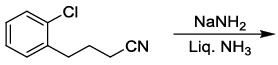Solution:
QUESTION: 6

The compound that contains the most acidic hydrogen is ​

Solution:
QUESTION: 7

The C-2 epimer of D-glucose is

Solution:
QUESTION: 8

The value of integral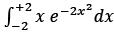is

Solution:
QUESTION: 9

ghe number of crystal systems and the number of Bravais lattices are, respectively,

Solution:
QUESTION: 10

For adsorption of a gas on a solid surface, the plot that represents Freundlich isotherm is (x = mass of gas, m = mass of adsorbent, P = pressure)

Solution:
QUESTION: 11

With respect to periodic properties, the CORRECT statement is

Solution:
QUESTION: 12

Which plot represents a spectrophotometric titration, where the titrant alone absorbs light in the visible region?

Solution:
QUESTION: 13

Among the following metal carbonyl species, the one with the highest metal-carbon back bonding is

Solution:
QUESTION: 14

The CORRECT order of Δo (the octahedral crystal field splitting of d orbitals) values for the following anionic metal complexes is

Solution:
QUESTION: 15

The decay modes of 14C and 14O are

Solution:
QUESTION: 16

Consider the following four xenon compounds: XeF2, XeF4, XeF6 and XeO3 The pair of xenon compounds expected to have non-zero dipole moment is

Solution:
QUESTION: 17

The CORRECT order of stability for the following carbocations is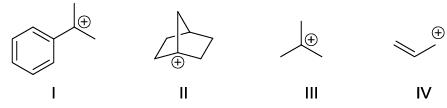Solution:
QUESTION: 18

Among the dimethylcyclohexanes, which one can be obtained in enantiopure form?

Solution:
QUESTION: 19

The major product formed in the following reaction is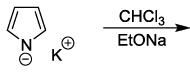Solution:
QUESTION: 20

The product X in the following reaction sequence is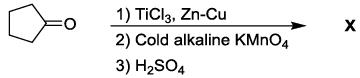Solution:
QUESTION: 21

The major product formed in the following reaction is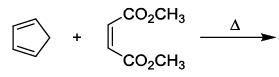Solution:
QUESTION: 22

The major products Y and Z in the following reaction sequence are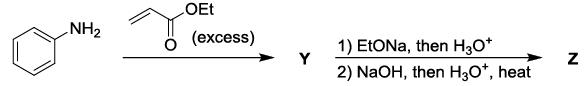Solution:
QUESTION: 23

The CORRECT order of carbonyl stretching frequencies for the following compounds is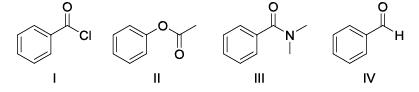Solution:
QUESTION: 24

The sequence of three steps involved in the following conversion is​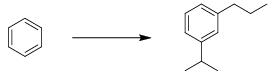Solution:
QUESTION: 25

The CORRECT expression that corresponds to reversible and adiabatic expansion of an ideal gas is

Solution:
*Multiple options can be correct
QUESTION: 26

The electrolyte AB2 ionises in water as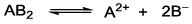The mean ionic activity coefficient (y± ) is

Solution:
QUESTION: 27

The reaction, A → Products, follows first-order kinetics. If [A] represents the concentration of reactant at time t, the INCORRECT variation is shown in

Solution:
QUESTION: 28

The behavior of Cl2 is closest to ideal gas behavior at

Solution:
QUESTION: 29

A vector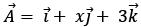is rotated through an angle and is also doubled in magnitude resulting in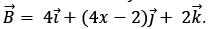An acceptable value of x is

Solution:
QUESTION: 30

With reference to the variation of molar conductivity (L) with concentration for a strong electrolyte in an aqueous solution, the CORRECT statement is

Solution:
*Multiple options can be correct
QUESTION: 31

Which of the following metal(s) is(are) extracted from its(their) sulfide ore(s) by self-reduction/air reduction method?

Solution:
*Multiple options can be correct
QUESTION: 32

In a saturated calomel electrode, the saturation is with respect to

Solution:
*Multiple options can be correct
QUESTION: 33

Consider the following six solid binary oxides: CaO, Al2O3, PbO, Cs2O, SiO2 and Sb2O3.The pair(s) of ionic oxides is(are)

Solution:
*Multiple options can be correct
QUESTION: 34

Choose the CORRECT answer(s) with respect to the magnesium-EDTA titration carried out in the pH range 7 – 10.5, using Solochrome black as indicator

Solution:
*Multiple options can be correct
QUESTION: 35

On reaction with NaNO2 and HCl, which of the following amino alcohol(s) will yield compound P?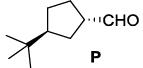Solution:
*Multiple options can be correct
QUESTION: 36

The CORRECT statement(s) about carbene is(are)

Solution:
*Multiple options can be correct
QUESTION: 37

The compound(s) that shows(show) positive haloform test is(are)

Solution:
*Multiple options can be correct
QUESTION: 38

Tetrapeptide(s) that gives(give) the following product on reaction with Sanger’s reagent followed by hydrolysis is(are)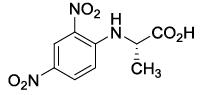Solution:
*Multiple options can be correct
QUESTION: 39

Which of the following set(s) of quantum numbers is(are) NOT allowed?

Solution:
*Multiple options can be correct
QUESTION: 40

The CORRECT expression(s) for isothermal expansion of 1 mol of an ideal gas is(are)

Solution:
*Answer can only contain numeric values
QUESTION: 41

The number of possible isomers for [Pt(py)(NH3)BrCl] is ______. (py is pyridine)The number of possible isomers for [Pt(py)(NH3)BrCl] is ______. (py is pyridine)

Solution:
*Answer can only contain numeric values
QUESTION: 42

The volume of 0.3 M ferrous ammonium sulphate solution required for the completion of redox titration with 20 mL of 0.1 M potassium dichromate solution is ______mL.

Solution:
*Answer can only contain numeric values
QUESTION: 43

Among the following hydrocarbon(s), how many of them would give rise to three groups of proton NMR peaks with 2:2:3 integration ratio?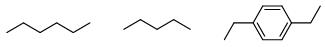Solution:
*Answer can only contain numeric values
QUESTION: 44

The number of stereoisomers possible for the following compound is ______.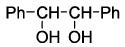Solution:

This is a FITB type of question.

It can be either RR or SS or RS. SR won’t be considered because it would be the same molecule as RS.

*Answer can only contain numeric values
QUESTION: 45

The number of hydrogen bond(s) present in a guanine-cytosine base pair is ______.

Solution:
*Answer can only contain numeric values
QUESTION: 46

The time for 50% completion of a zero order reaction is 30 min. Time for 80% completion of this reaction is ______ min.

Solution:
*Answer can only contain numeric values
QUESTION: 47

Consider the reaction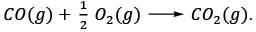The value of ΔU for the reaction at 300 K is K is 281.8 kJ mol-1. The value of DH at same temperature is ______ kJ mol-1 (rounded up to the first decimal place).
[R = 8.3 J K-1 mol-1]

Solution:
*Answer can only contain numeric values
QUESTION: 48

The nuclear spin quantum number (I) of a nucleus is 3/2. When placed in an external magnetic field, the number of possible spin energy states it can occupy is ______.

Solution:
*Answer can only contain numeric values
QUESTION: 49

The value of Cv for 1 mol of N2 gas predicted from the principle of equipartition of energy, ignoring vibrational contribution, is ______ J K–1 mol–1 (rounded up to two decimal places). [R = 8.3 JK–1mol–1]

Solution:
*Answer can only contain numeric values
QUESTION: 50

Assuming ideal gas behavior, the density of O2 gas at 300 K and 1.0 atm is ______ g L–1 (rounded up to two decimal places).
[R = 0.082 L atm mol–1 K–1, molar mass of O2 = 32]

Solution:
*Answer can only contain numeric values
QUESTION: 51

How many of the following interhalogen species have 2 lone pairs of electrons on the central atom?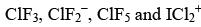Solution:
*Answer can only contain numeric values
QUESTION: 52

24Na decays to one-fourth of its initial amount in 29.8 hours. Its decay constant is_________ hour–1 (rounded up to four decimal places).

Solution:
*Answer can only contain numeric values
QUESTION: 53

The magnitude of crystal field stabilization energy (CFSE) of octahedral [Ti(H2O)6]3+ complex is 7680 cm−1. The wavelength at the maximum absorption (λmax) of this complex is __________ nm (rounded up to the nearest integer).

Solution:
*Answer can only contain numeric values
QUESTION: 54

Elemental analysis of an organic compound containing C, H and O gives percentage composition: C: 39.9 % and H: 6.7 %. If the molecular weight of the compound is 180, the number of carbon atoms present in the molecule is________.

Solution:
*Answer can only contain numeric values
QUESTION: 55

The number of compounds having S-configuration among the following is _________.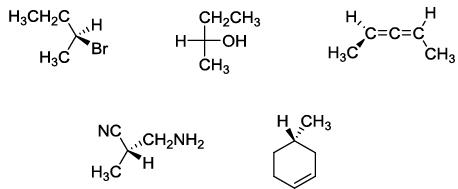Solution:
*Answer can only contain numeric values
QUESTION: 56

The emf of a standard cadmium cell is 1.02 V at 300 K. The temperature coefficient of the cell is –5.0 x 10–5 V K–1. The value of ΔH° for the cell is _________ kJ mol-1 (rounded up to two decimal places).
[1 F = 96500 C mol-1]

Solution:
*Answer can only contain numeric values
QUESTION: 57

For the reaction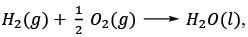the following information is given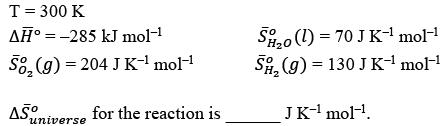Solution:
*Answer can only contain numeric values
QUESTION: 58

For H2 molecule, the fundamental vibrational frequency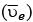can be taken as 4400 cm-1. The zeropoint energy of the molecule is ______ kJ mol-1 (rounded up to two decimal places).
[h = 6.6×10-34 J s, c = 3×108 m s-1,NA = 6×1023 mol-1]

Solution:
*Answer can only contain numeric values
QUESTION: 59

The solubility of PbI2 in 0.10 M KI(aq) is ______ ×10–7 M (rounded up to two decimal places).
[The solubility product, sp = 7.1×10–9]

Solution:
*Answer can only contain numeric values
QUESTION: 60

The electron of a hydrogen atom is in its nth Bohr orbit having de Broglie wavelength of 13.4 Å.
The value of n is ______ (rounded up to the nearest integer).
[Radius of nth Bohr orbit = 0.53n2 Å, π = 3.14]

Solution: